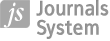About two diagnistic strategies of mathematical models in economics based on the statistical distribution analysis of residal errors

Więcej
Ukryj
 1 Alcide De Gasperi University of Euroregional Economy in Józefów Poland, Warsaw
Data publikacji: 30-09-2017

JoMS 2017;34(3):269–276

SŁOWA KLUCZOWE
STRESZCZENIE
Two diagnostic methods of mathematical models in economic studies that are based on the statistical analysis of residual errors O-S (Observation-Calculation) are considered in the article. The first method is recommended for sample sizes n within 30 < n < 500, using the x-test to verify the normality of the O-C values. The second method is recommended for the diagnosis of O-C errors with the volume n > 500. The famous Cambridge professor H. Jeffreys made the conclusion that such samples usually follow the Pearson distribution of type VII, which has a positive kurtosis. The mathematical model for volumes of O-C values n > 500 is considered adequate if the kurtosis for O-C is in the limits of 6,0-1,2 with insignificant asymmetries. The model is acceptable if the kurtosis for O-C is in the limits of 1,2-0,0. The model is considered inadequate (unacceptable) if the errors of O-C have a significant negative kurtosis or significant asymmetry.

REFERENCJE (14)
1.
Cramer, H. (1946). Mathematical methods of statistics, Princeton: University Press.

2.
Dzhun, I.V. (2011). Method for diagnostics of mathematical models in theoretical astronomy and astrometry, ‟Kinematics and Physics of Celestial Bodies”, vol. 27, № 5, p. 260–264, Allerton Press, Inc.

3.
Dzhun, I.V. (2015). Neklasichskaya teoriya pogreshnostey izmereniy [Non-classical Error Theory in Measurement] Rivne, Estero Publ., p. 168.

4.
Dzhun, I.V. (2012). What are differences “observation-calculation” bound to be during modern experiments in astrometry, ‟Kinematics and Physics of Celestial Bodies”, vol. 28, № 1, p. 70–78, Allerton Press, Inc.

5.
Gauss, C.F. (1809). Theoria motus corporum coelestium in sectionibus conicis Solem ambientium, Hamburgi: Frid. Pethers et I. H. Besser.

6.
Geary, R.C. (1936). Distribution of student’s ratio for non-normal samples, ‟Journal of the Royal Statistical Society”, suppl. 3.

7.
Hampel, F.R., Ronchetti, E.M., Rousseeuw, P.J., Stahel, W.A. (1985). Robust statistics, The Approach Based on Influence Functions, John Wiley & Sons.

8.
Jeffreys, H. (1939). The law of errors in the greenwich variation of latitude observations, ‟Mon. Not. of the RAS”, vol. 99, № 9, p. 703–709.

9.
Jeffreys, H. (1940). Theory of probability, Sec. Eddition, Oxford, p. 468.

10.
Lukacs, E.A. (1942). A characterization of the normal distribution, ‟Annals of Mathematical Statistics”, vol. 13, № 91.

11.
Newcomb, S. (1886). Generalized theory of the combination of observations so as to obtain the best result, ‟Amer. J. Math” № 1/14, p. 1–249.

12.
Pearson, K. (1902). On the mathematikal theory of errors of judgment with special reference to the personal equation, ‟Philosophical Transactions of the Royal Society of London” Ser. A, vol. 198, p. 253–296.

13.
Stigler, S.M. (1975). Contribution to the discussion of the meeting of robust statistics, [in:] Proceedings of the 40th Session of the ISI, Warsaw. – Bull. Int Statist. Inst., v. XLVI, book 1, p. 383–384.

14.
Student (1927). Errors of routine analysis, ‟Biometrika” vol. 19, p. 151–164.

 eISSN: 2391-789X ISSN: 1734-2031